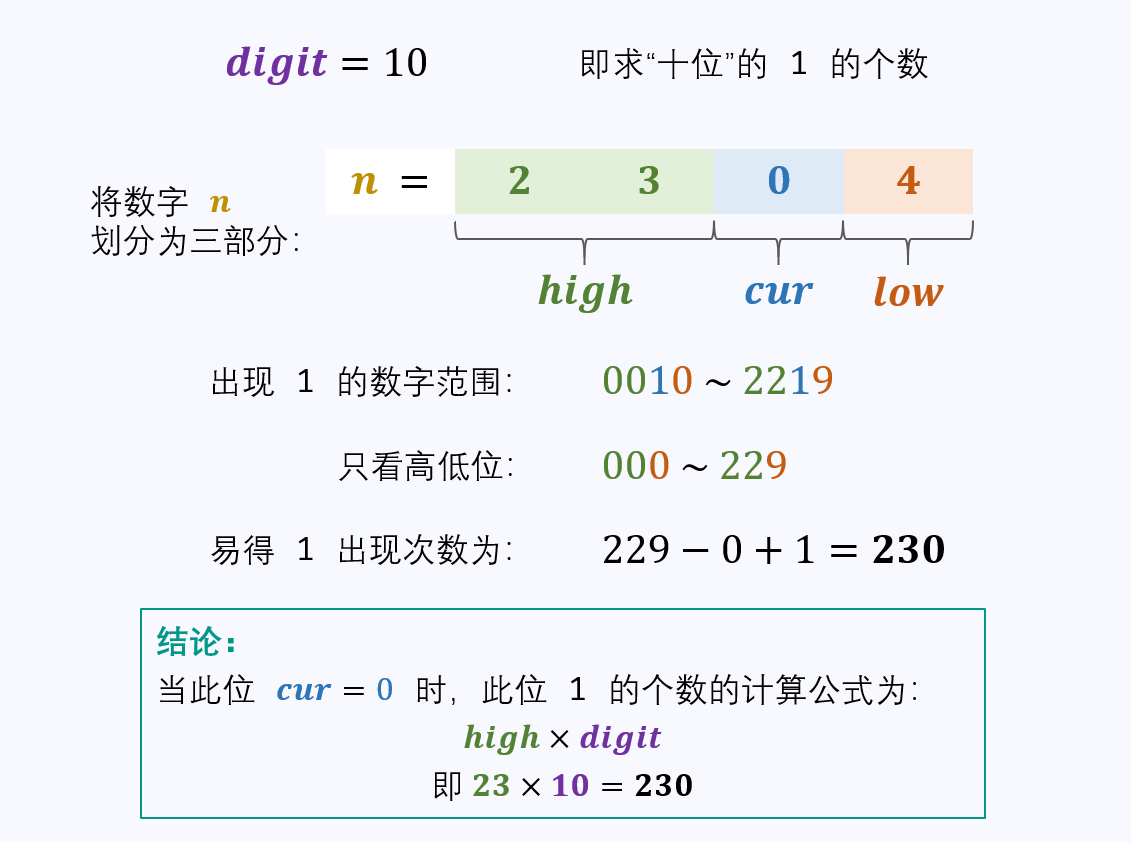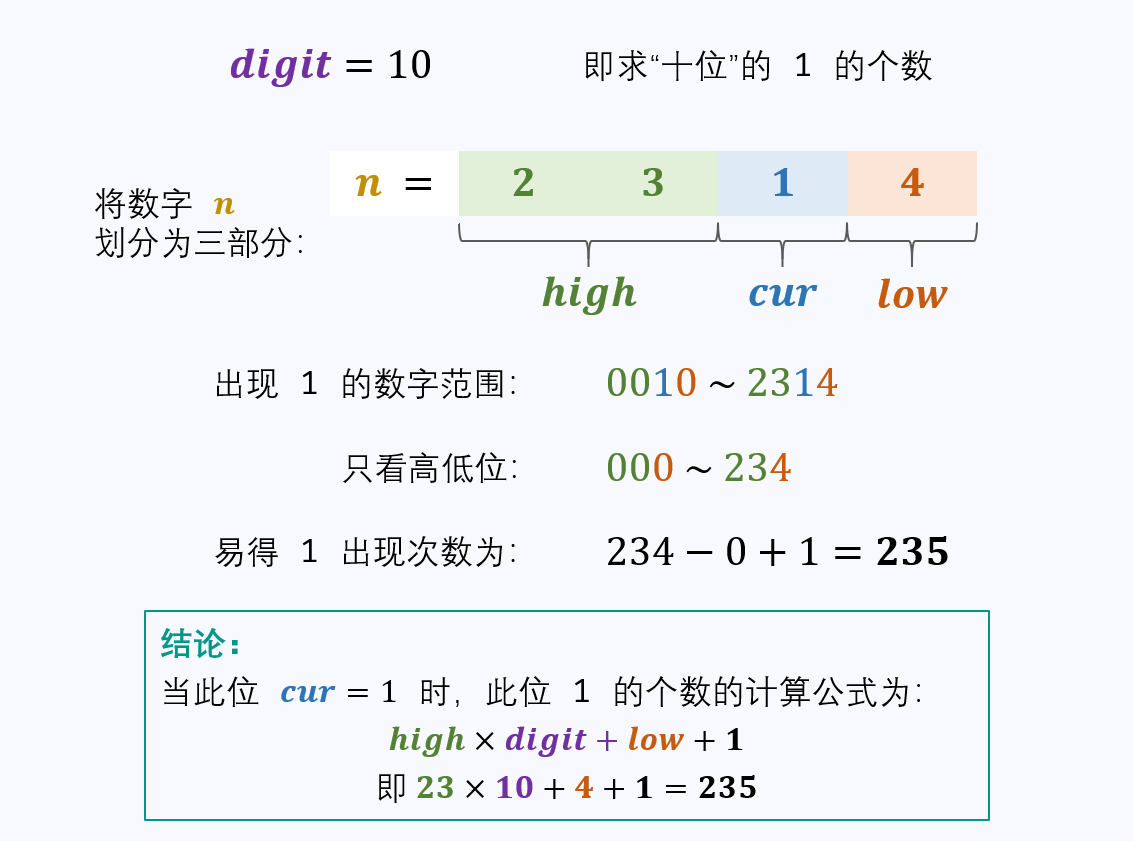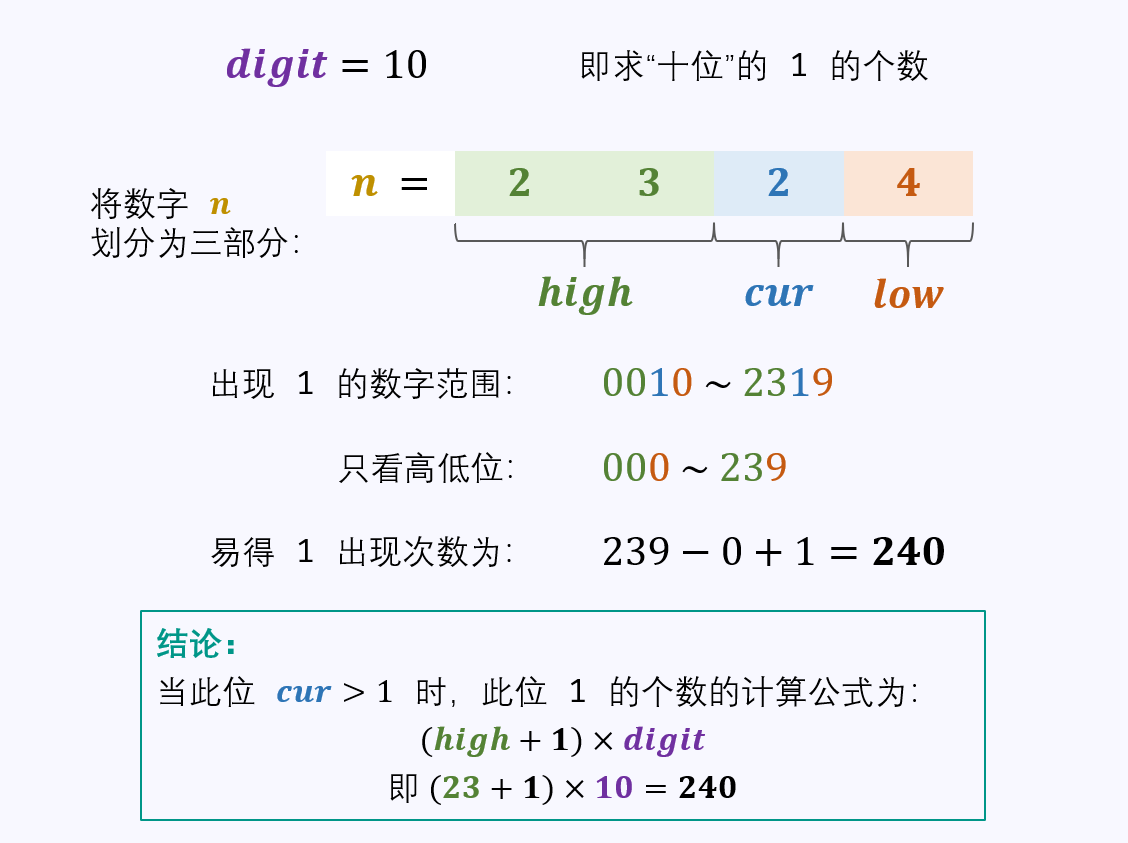# 1、1到n整数中1出现的次数

输入：n = 12



输入：n = 13



## 1.1、解题思路## 1.2、算法

    public int countDigitOne(int n) {
//digit = 1 表示个位
int digit = 1, res = 0;
//high：高位（1-10）初始进来是0
int high = n / 10;
//cur：初始进来 就是个位数
int cur = n % 10;
//low：低位为0
int low = 0;
/** high ==0 的时候可能是首次进入，name这个时候cur就不能等于0 */
/** cur ==0 的时候可能是首次进入hign就不能等于0 */
/** 也就是说必须有一个不等于0 */
while (high != 0 || cur != 0) {
if (cur == 0) {
res += high * digit;
} else if (cur == 1) {
res += high * digit + low + 1;
} else {
res += (high + 1) * digit;
}

//开始走下一个
low += cur * digit;
cur = high % 10;
high /= 10;
digit *= 10;
}
return res;
}


## 1.3、测试


@Test
public void test(){
System.out.println(countDigitOne(3412));
}